# Matrices and Absolute Value Chapter Exam

Exam Instructions:

Choose your answers to the questions and click 'Next' to see the next set of questions. You can skip questions if you would like and come back to them later with the yellow "Go To First Skipped Question" button. When you have completed the practice exam, a green submit button will appear. Click it to see your results. Good luck!

Answered 0 of 30

### Page 1

#### Question 4 4. Which graph matches the equation below?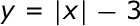### Page 2

#### Question 7 7. Find the determinant of the matrix.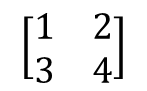#### Question 9 9. Which graph matches the equation below?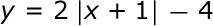### Page 3

#### Question 11 11. What is the correct equation for the function shown?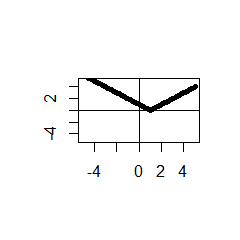### Page 4

#### Question 17 17. Which system of equations is represented by the following matrix? (Think of the vertical line as the equal sign.)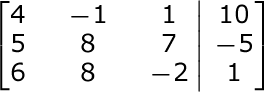#### Question 20 20. Find the determinant of the following matrix.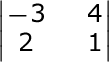### Page 5

#### Question 23 23. Which equation matches the graph below?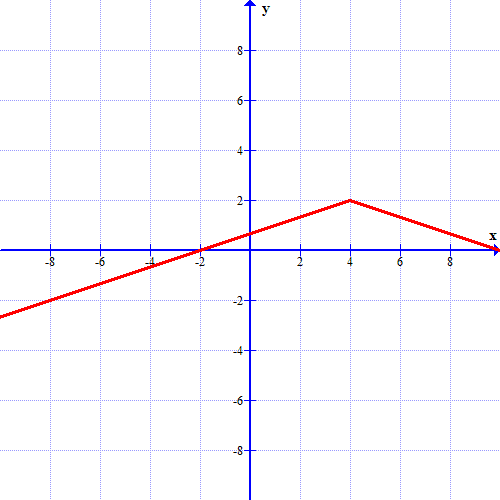### Page 6

#### Question 29 29. What is the equation for the graph shown?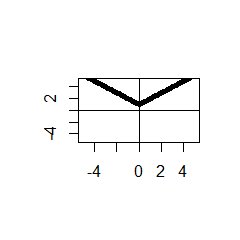#### Matrices and Absolute Value Chapter Exam Instructions

Choose your answers to the questions and click 'Next' to see the next set of questions. You can skip questions if you would like and come back to them later with the yellow "Go To First Skipped Question" button. When you have completed the practice exam, a green submit button will appear. Click it to see your results. Good luck!

Support